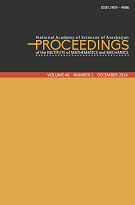P R O C E E D I N G S
of the
Institute of Mathematics and Mechanics

 ISSN 2409-4986 (Print) ISSN 2409-4994 (Online)

## Volume 42, Issue 1

On solvability of an external problem with impedance boundary condition for Helmholtz equation by integral equations method
by Rahib J. Heydarov
39
A variation of the \$L^p\$ uncertainty principles for the Fourier transform
by Fethi Soltani and Jaber Ghazwani
1024
Trigonometric approximation in weighted Orlicz spaces
by Yunus Emre Yildirir and Ramazan Cetintas
2537
Lie derivatives of almost contact structure and almost paracontact structure with respect to \$X^{V}\$ and \$X^{H}\$ on tangent bundle \$T(M)\$
by Haşim Çayır
3849
A new constructive method for solving of bisingular integral equations with Cauchy kernel
5066
On smooth solutions of a class of boundary value problems on a finite segment for second order equations with operator coefficients
6772
On asymptotic behavior of local probabilities of nonlinear boundary crossing by a random walk
by Fada G. Rahimov, Tarana E. Hashimova and Vugar S. Khalilov
7380
Multilinear fractional integral operators with rough kernel on modified Morrey spaces
by Elmira A. Gadjieva, Amil A. Hasanov and Zaman V. Safarov
8192
Formula for second regularized trace of the Sturm-Liouville equation with spectral parameter in the boundary conditions
by Hajar F. Movsumova
93105
Necessary conditions of Riesz property of root vector-functions of Dirac discontinuous operator with summable coefficient
by Leyla Z. Buksayeva
106115
Global bifurcation of a second order nonlinear elliptic problem with an indefinite weight function
by Ziyatkhan S. Aliyev and Shanay M. Hasanova
116125
Approximation of analytic functions by sequences of linear operators in a polydisc
by Rashid A. Aliev
126133
Outstanding mathematician and pedagogue (To the 100-th anniversary of Majid Rasulov) 134138
 © 2022 Institute of Mathematics and Mechanics